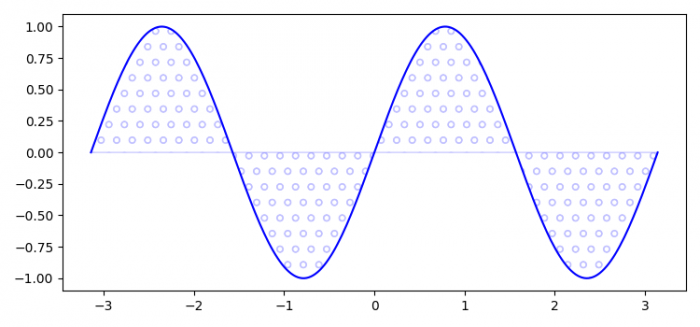# How do I fill a region with only hatch (no background colour) in matplotlib 2.0?

To fill a region with only hatch (no background color) in matplotlib, we can take the following steps −

## Steps

• Set the figure size and adjust the padding between and around the subplots.

• Initialize a variable n to store the number of sample data.

• Create a figure and a set of subplots.

• Plot the x and y data points.

• Fill the area between x and y with circle hatches, edgecolor="blue".

• To display the figure, use show() method.

## Example

import numpy as np
import matplotlib.pyplot as plt

# Set the figure size
plt.rcParams["figure.figsize"] = [7.50, 3.50]
plt.rcParams["figure.autolayout"] = True

# Number of sample data
n = 256

# x and y data points
x = np.linspace(-np.pi, np.pi, n, endpoint=True)
y = np.sin(2 * x)

# Figure and set of subplots
fig, ax = plt.subplots()

# Plot the data points
ax.plot(x, y, color='blue', alpha=1.0)

# Fill the area between the data points
ax.fill_between(x, y, color='blue', alpha=.2, facecolor="none", hatch="o", edgecolor="blue", linewidth=1.0)

# Display the plot
plt.show()

## Output

It will produce the following output −